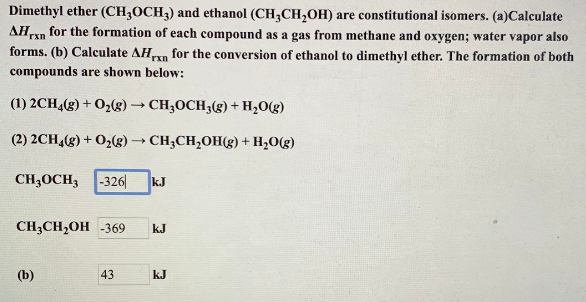# Dimethyl ether (CH3OCH3) and ethanol (CH3CH2OH) are constitutional isomers. (a) Calculate ΔHrxn for the formation of each compound as a gas from methane and oxygen; water vapor also forms. (b) Calculate ΔHrxn for the conversion of ethanol to dimethyl ether. The formation of both compounds are shown below: (1) 2CH4(g) + O2(g) → CH3OCH3(g) + H2O(g) (2) 2CH4(g) + O2(g) → CH3CH2OH(g) + H2O(g)